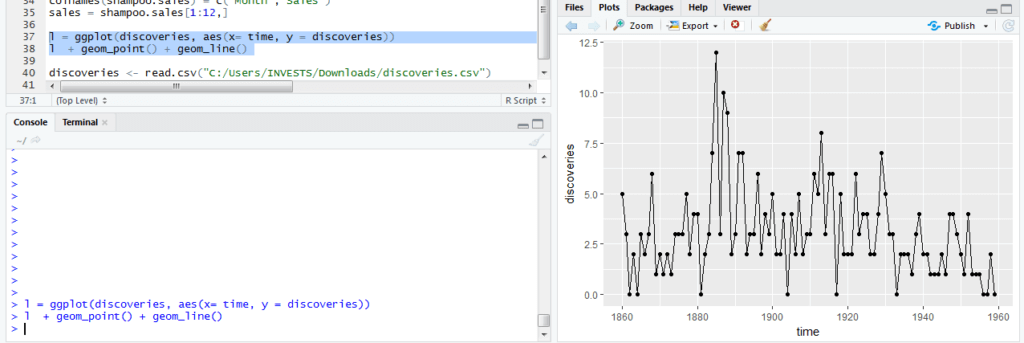# Master Data Vizualization using R Programming Language with ggplot2

0
1924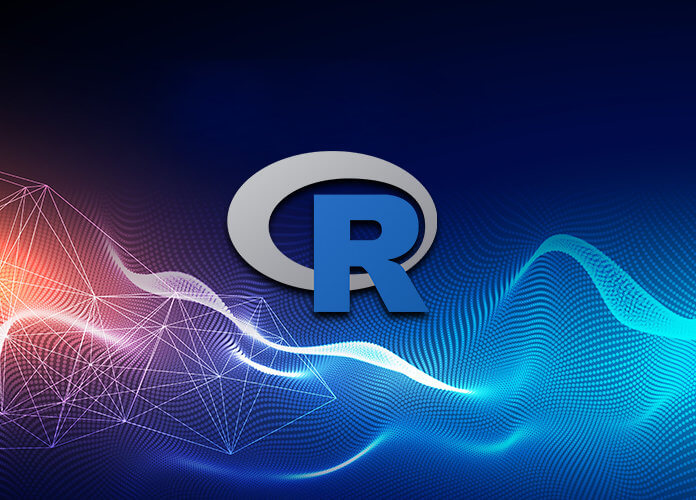Data visualization is a technique of summarizing data in a graphical or pictorial approach. With a visual presentation, it is easy to identify relationships, trends and patterns present in the data. Such information cannot be obtained from raw data. Important elements in data visualization include having good quality data, selecting the right data, designing the chart and sharing it with relevant stakeholders. In R, there are data visualization capabilities in base R and in dedicated packages. A detailed description of data visualization tools is available here http://cran.r-project.org/web/views/Graphics.html. The package ggplot2 developed by Hadley Wickham has become the preferred approach to data visualization.

As an R package, ggplot2 is an implementation of Lee Wilkinson’s grammar of graphics which emphasizes on building graphs using independent elements. The elements are described below:

• The data that will be visualized
• A set of aesthetic mappings that describe mapping of data to aesthetic attributes
• Geometric objects that will be shown on the plot for example lines or points
• Statistical transformations that will summarize the data for example counts. These transformations are not mandatory but they are useful for including additional information
• The scales that show a legend or axes that aid interpretation of data
• A faceting that shows how data subsets will be divided and displayed

To use ggplot2 for visualization the data is required to be in a data frame. This restriction is in place to ensure ggplot2 is only used for visualization and not data manipulation. To demonstrate creation of graphs we will use this dataset https://www.kaggle.com/uciml/pima-indians-diabetes-database/data provided by kaggle. The file contains data on characteristics of diabetic patients.

```#read in the csv data
diabetes\$Outcome = as.factor(diabetes\$Outcome)
diabetes\$Outcome = factor(diabetes\$Outcome,labels = c('Not diabetic','Diabetic'))
str(diabetes)

To describe mapping of data to axes, the aes function is used. In the aes function, we specify the variable that will be mapped to x-axis, the variable that will be mapped to y-axis and the colors to be used in plotting. All the variables specified in aes are required to be part of the plot thus guaranteeing a ggplot object can be stored and reused. An example is shown below where BMI will be placed on the x-axis and blood pressure will be placed on the y axis.
aes(x=BMI,y=BloodPressure)
Setting aesthetic mappings can be done together with the other graph elements and there is flexibility of modifying the mappings later using “+ “notation. An example of this notation is shown below where points are added to the plot.

```p = ggplot(diabetes,aes(x=BMI,y=BloodPressure)) + geom_point()
p```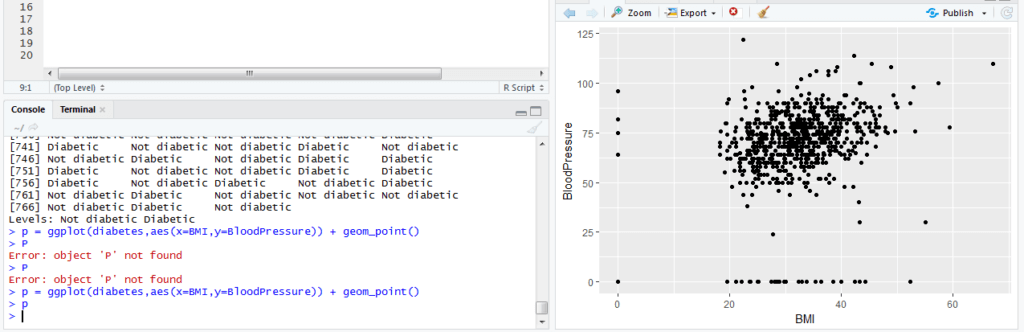The default aesthetics can be changed, overridden or removed when adding layers. For example, to change the color of points to blue the code below is used.

`p + geom_point(colour = 'darkblue')`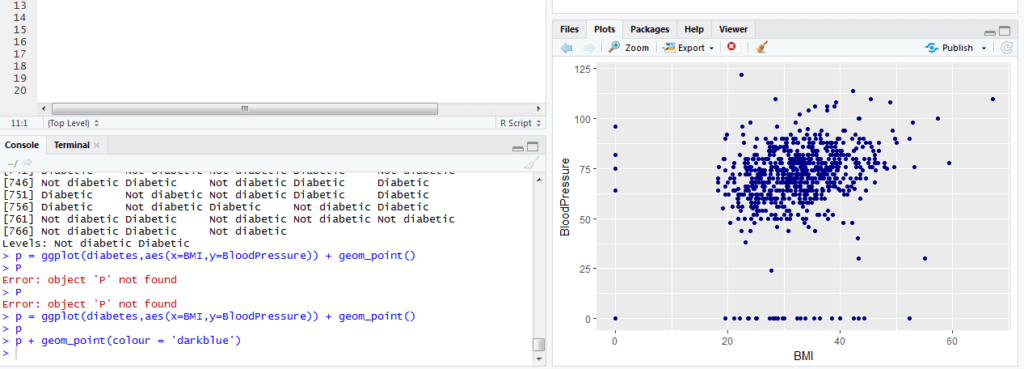Visualization of geometric groups is supported through specifying a factor variable. The combination of variables specified in group is used and when this does not provide satisfactory results the interaction option can be used to specify grouping.

There is a wide range of geometric objects available in ggplot2 which are exhaustively described here http://ggplot2.tidyverse.org/reference/#section-layer-geoms. All commonly used graphs such as scatterplots, histograms, bar charts and box plots are available. Statistical functions are also described in the documentation. These functions create new variables that can be used instead of untransformed variables.
In the previous section, we focused on building blocks off ggplot visualizations. In the next section, examples will be used to demonstrate how the building blocks are combined.
A bar chart is a visualization that is used to compare categories. This comparison is possible because the heights of the bars represent a quantity. To demonstrate plotting bar charts let us use data on endangered species available here https://www.kaggle.com/cites/cites-wildlife-trade-database
The data is loaded using the code below:

`cites.data = read.csv("C:/Users/INVESTS/Downloads/comptab_2018-01-29 16_00_comma_separated.csv/comptab_2018-01-29 16_00_comma_separated.csv")`

We would like to compare different purposes of trading in endangered species. The code used is shown below:

```g = ggplot(cites.data,aes(Purpose))
g + geom_bar()+ coord_flip() +
xlab("Purpose of trade") + ylab("Count") +
ggtitle("Comparison of different purposes of trade in CITES") +
theme_bw()```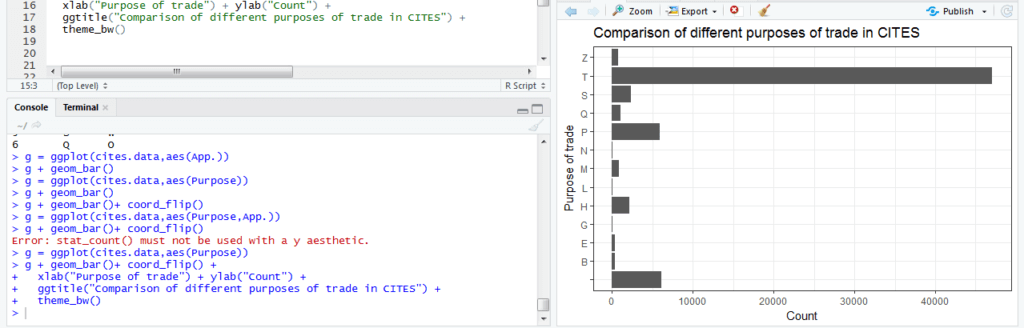A histogram is a visualization that aids understanding of the distribution of a continuous variable. A histogram shows skewness, outliers and the range of observations. The most important parameter in plotting a histogram is the bin which controls width of columns. To demonstrate plotting of a histogram blood pressure of diabetic patients will be used

The code used to plot a histogram is shown below. A density and a line showing the mean are included.

```h = ggplot(diabetes,aes(x = BloodPressure)) + geom_histogram(aes(y=..density..), colour="black") +
geom_vline(aes(xintercept=mean(BloodPressure)),color="blue", linetype="dashed", size=1) +
geom_density(alpha=.2, fill="#FF6666")
h```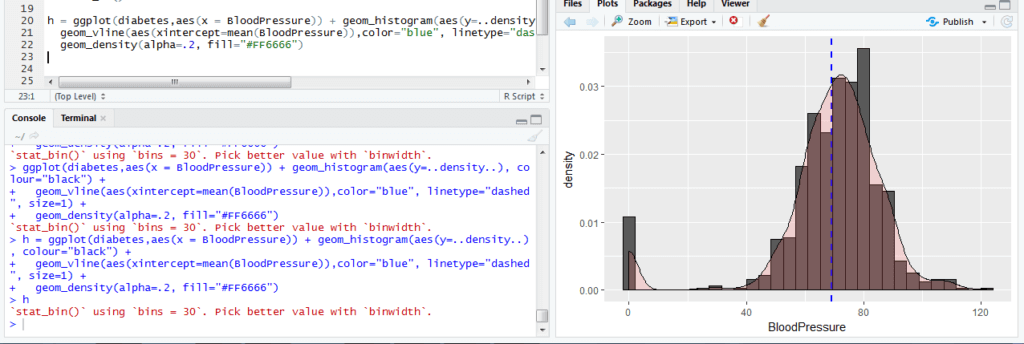A boxplot is used to visualize continuous data by presenting the maximum, minimum, median and observations considered outliers. A box plot of blood glucose of diabetes patients will be created. The observations are plotted and outliers are shown in red. The code used is shown below.

```b = ggplot(diabetes, aes(y = Glucose, x=Outcome))   + geom_boxplot(outlier.colour = "red", outlier.shape = 1)  + geom_jitter(width = 0.2)
b```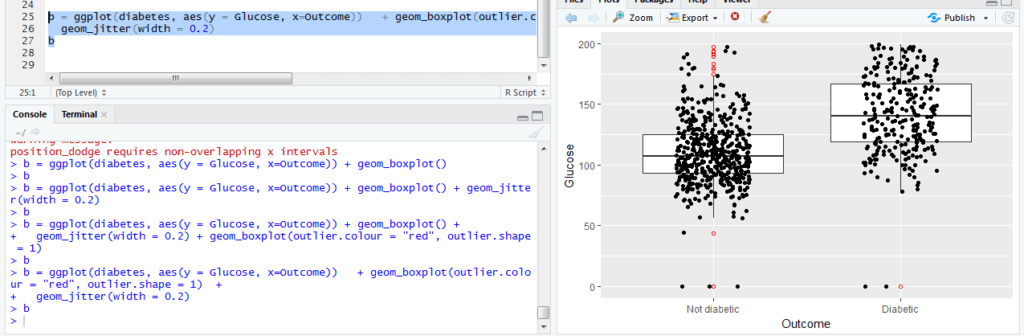A scatter plot is used to visualize the relationship between two continuous variables. We will plot blood pressure and the BMI of diabetic patients. Observations will be colored differently for diabetic and non-diabetic patients. The code used is shown below

```s = ggplot(diabetes, aes(x = BloodPressure,y = BMI,color = Outcome, shape = Outcome))
s + geom_point() +
geom_smooth(method=lm, se=FALSE, fullrange=TRUE)```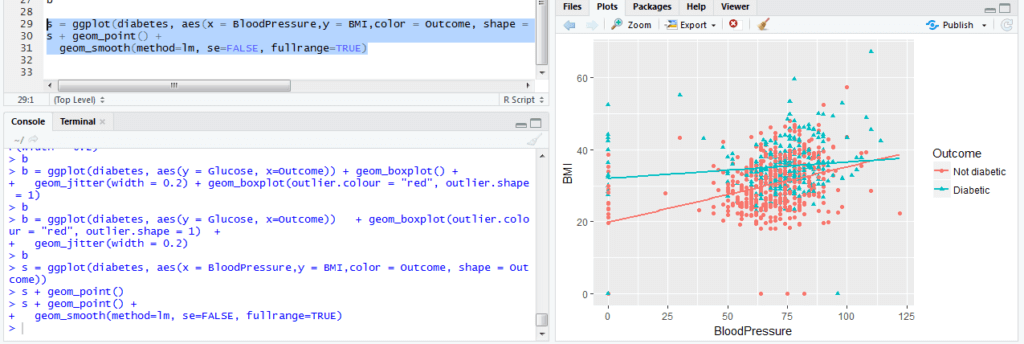A line plot is useful for visualizing trends in data over a period of time. To demonstrate creation of line plots we will use number of discoveries data available here http://www-eio.upc.edu/~pau/cms/rdata/datasets.html
```l = ggplot(discoveries, aes(x= time, y = discoveries))